# How to add a point to the highest point of a Bezier curve

Hello, I would like to ask how to add a point to the highest point of the Bezier curve through a python script. Thank you so much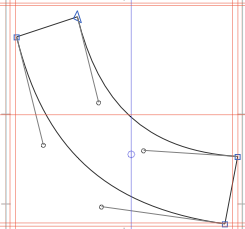Because it is not a convex function, it cannot be implemented using the method of adding extreme points

For example, a convex function can add the highest point by adding an extreme point, as shown in the following figure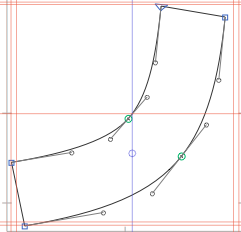That is not an extreme point. That would be a point at edge of the bounding box. You might mean a point where the curvature is the highest.

The GSPathSegment class has a `curvature` method that will return a ist of points with high curvature.

``````path = Layer.shapes
segments = path.segments
for s in segments:
print("curvature", s.curvature())
``````

The return value is not a list of points but the “t” value and the curvature (packaged in an NSPoint).

1 Like

Thank you very much ！！！

How to resolve this error: ‘RSegment’ object has no attribute ‘curvature’

Where and how are you running the code?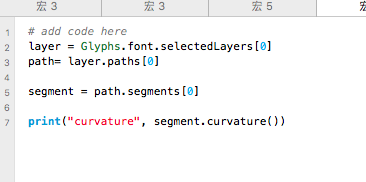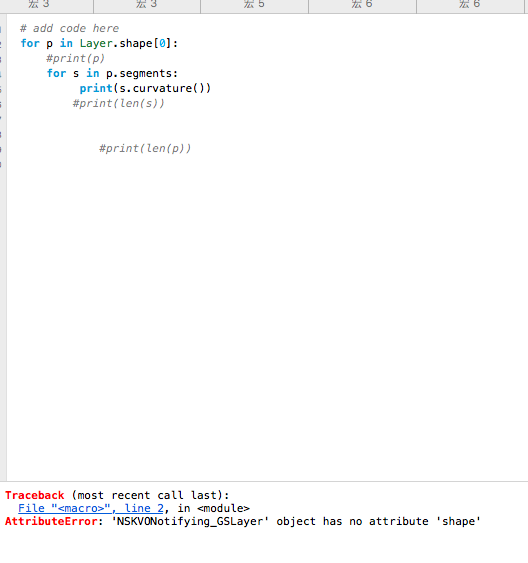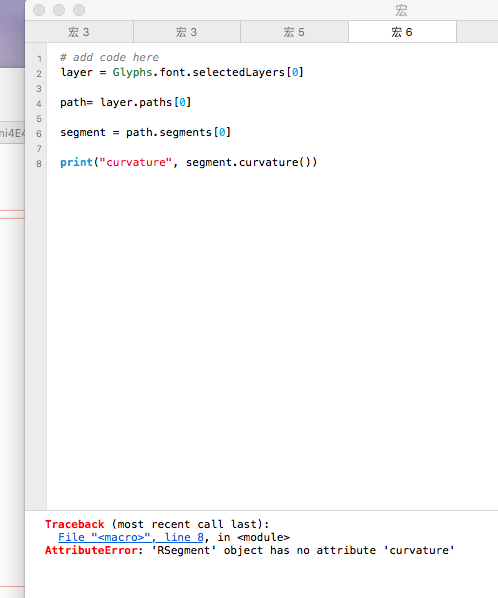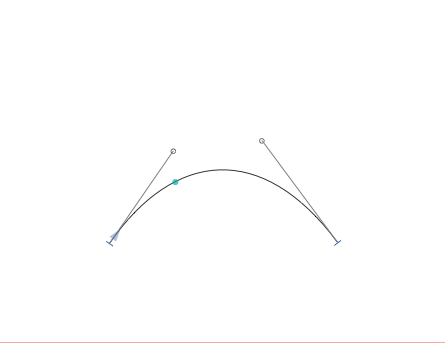That is not implemented in Glyphs 2. Sorry.

Thanks very much，this is a version issue，I’ve already solved it。ButHow can I obtain the point coordinates corresponding to the maximum curvature value through the curvature value and add them to the Bezier curve。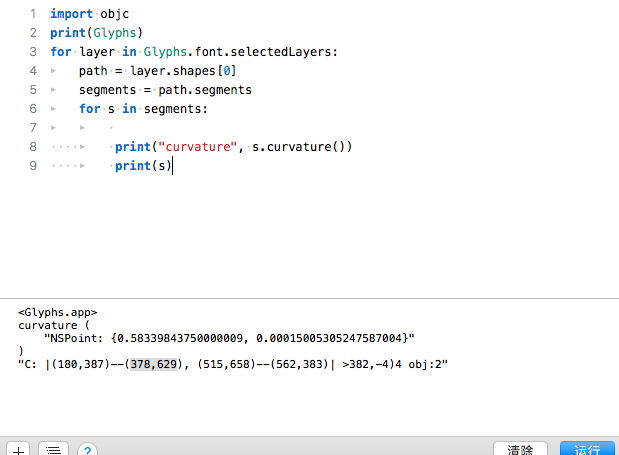The value stored in s is the value of the node, not the point on the Bezier curve

When you get the value from `s.curvature()`, you can insert the point as described in the other thread.

``````for layer in Glyphs.font.selectedLayers:
path = layer.shapes
segments = path.segments
newSegments = []
for s in segments:
c = s.curvature()
if len(c) > 0:
length = s.length()
t = c.x
maxC = c.y
if length * length * maxC > 5: # the 5 is a arbitrary number I picked her.
split = s.splitAtTime_firstHalf_secondHalf_(t, None, None)
newSegments.extend(split)
continue
newSegments.append(s)
print("__newSegments", newSegments)
path.segments = newSegments
path.checkConnections()
``````

The value returned by newSegments is only the value of the node, not the point at the most curved part between the two nodes on the curve. I want to obtain the point at the most curved part of the curve, such as the red point on the curve in the following figure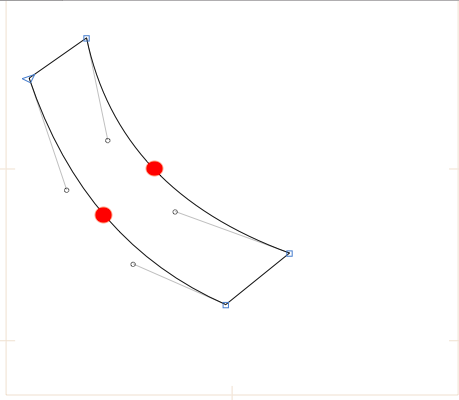As shown in the following figure, the most curved point on the curve is the highest point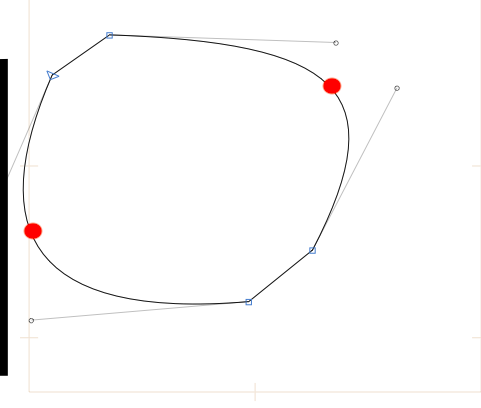I think it can be judged by the distance from a point on the curve to the two endpoints of the curve, but I won’t write this part of code

The maximum curvature can be at different places of the curve.
Just one example: